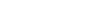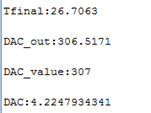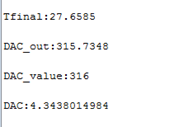# 4-20mA current loop transmitter: Arduino code

Good morning,

I would like to have some explanations concerning the arduino code for 4-20mA current loop transmitter.
In the code example, you send to the DAC the value 64:

/// at DAC value 290 the current output will be around 4mA and
///at DAC value 1500 the current output will be around 20mA
/// you can change these values to tune the 4-20mA output

Why have you choosen 290 and 1500 as DAC values and not another combination?

Why do you multiply i by 0.013?
Serial.println(i*0.013);

Hi,
In this code we are using the 4-20mA Tx in following configuration

1. Vref 5V
2. Resolution 12 bit
Thats why we chose the values 290 and 1500.
at 4mA the DAC value is around 290, using these two we can calculate the multiplication factor
multiplication factor = 4/290 == 0.013
If you want better resolution you can use it with following config
3. set the vref to 2.048V ( using on board gain jumper)
4. Resolution 16 bit

Thanks

Ok, Thanks.

The DAC is 12bit that corresponds to 4096 voltage levels, then I can determine the resolution by the formula: LSB=Vref/2^12
…but I am still missing how do you determine that @4mA the DAC value 290.

the XTR 115 outputs 0.25mA to 25mA and its linear output.
its vref is 2.5V, based on these 3 values we can calculate the voltage value where it will output 4mA. using that voltage value we can calculate the DAC value.
and the other way to do is this
i use a 4-20mA meter to measure the output and start changing the dac values from 0 to 4096 and i note down the dac values when the current is 4mA and when it is 20mA. This saves me a lot of time in calculations.

Thanks

Now I better understand.
On the basis of the XTR 115 outputs I obtain that the voltage value for 4mA is 0.4V, then using use the following formula:

Vrefdac/4096 = 0.4/X where X = 0.4V*4096/5V= 328

I obtain the DAC values: 328 for 4mA and 1650 for 20mA

They are quite different form yours, so I was wondering if I still missing something.

I am just asking this question because I have to pass a float value to the DAC.
I have casted the float in 4 bytes but now I am a little bit stuck in writing them to the DAC following the example code:

for (int i=290; i <= 1500; i++)
{
Wire.beginTransmission(0x60 );
Wire.write(64);
// Wire.write(64);
Wire.write(i >> 4); // 8 MSB
Wire.write((i & 15) << 4); //4 LSB
delay(100);
Serial.print(“4-20mA current transmitter output “);
Serial.println(i*0.013);
Serial.print(”\n”);
Wire.endTransmission();
}

Any suggestion will be really appreciated.

do you have any example value you want to pass to the DAC.
Thanks

From my sensor I will send temperatures values: the sensor measures from 20°C to 150°C.
So I can send 25.4367, 59.5436, 120.3456, etc…

In the case of 25.4367, I calculate the value to pass:

Output value= T value*4095/150= 694.42191

Is it correct? In this case which is the right rounded value to guarantee the exact correspondence to the measured temperature?

we can not send these kind of float values but we generate float value output by sending raw byte values
so for example lets say when you send 328 it will output 4mA and now if you send 329 it will change the output value to 4.01219mA. the values will increase by a 0.01219 factor when you increase the count value by one.

I think there was a misunderstanding.

I purchased the board because my idea was to convert a temperature signal measured via Arduino in 4-20mA
Attached you can find the block diagram of my system.

I would like to pass the temperature signal (float) coming from Arduino to the DAC, convert it to DAC level and obtain the correspondent signal in mA.

For example,
T=25.4367
Output value= T value*4095/150= 695

But 695 doesn’t correspond to something around 4mA. For this reason I am filling a little bit lost…

got it.
Yes, you can do it. this is how it needs to be done
read the temp using the arduino. lets say for example when temp is 25’C arduino raw value is 220 and when the temp is 150’C the arduino raw value is 2500.
now you can pass these values on the DAC and you will know that when DAC reads x mA it means temp is 25 'C and when it y mA the temp is 150’C.

and rather then converting raw bytes into temp and then try to send it over to DAC and convert it back to temp. send raw values from temp sensor to dac.

in this example i am readings an analog potentiometer and converting it into 4-20mA output.

Ok.
Thanks to your example, it is more clear to me what I have to do.

The statements:

• 25’C arduino raw value is 220
• 50’C the arduino raw value is 2500.
are still not so clear to me. How do you determine the raw values?

The communication between Arduino and the sensor is via RS485 half duplex and the protocol is Modbus RTU. I read directly the pressure values from the UART, the information is 4 bytes that I cast into float.
I don’t figure out how to pass the the floats values to the DAC of the transmitter’s board.

if its a 4 byte value it means its a 32bit value, you wont be able to set a 32 bit value in a 12bit dac.
You will need to convert this 32bit value into 12bit value ( bit shift is an easy way to do this). By doing this you will loose some accuracy but by looking at the example values you mentioned earlier (T=25.4367) it wont be a terrible loss.
then you will need to get the 4-20mA output and temp readings at 2 set points to calculate any new value. this will be linear equation.
In this tutorial we are doing just opposite of what you are trying to do

Thank you for the link. I will have a look to the tutorial. .
Basically my sensor can measure from 25°C to 150°C, in your board at 290 the current output is 4mA and at 1500 the current output is 20mA.
If I measure 25.4367, I will do like that:

Setpointmin= 25
Setpointmax=150
Outmin=290
Outmax=1500

DAC_value= (25.4367-Setpointmin)/(Setpointmax-Setpointmin)*(Outmax-Outmin)+Outputmin=294.2272

I will round this value and I will write it to the DAC using your example.

Do you think it should work?

Good morning,

I think I still need some help because I am missing something…
Below you can read a part of my program

void loop()
{
delay(1000);
modbus_update();

digitalWrite(LED, HIGH);
delay(100);
digitalWrite(LED, LOW);

//Shift and Cast value temperature to float

Tfinal =c* Thead3 + d * Thead2 + e * Thead + f; //Calcultion of final temperature

DAC_out= ((Tfinal-Setpointmin)/(Setpointmax-Setpointmin))*(Outmax-Outmin)+Outmin;
DAC_value= round(DAC_out);

``````for (int i=290; i <= 1500; i++)
{
Wire.beginTransmission(0x60);
Wire.write(DAC_value);
Wire.write(i >> 4);        // 8 MSB
Wire.write((i & 15) << 4); //4 LSB
delay(1000);
Serial.print("4-20mA current transmitter output ");
Serial.println(i*0.013);
Serial.print("n");
Wire.endTransmission();
}
``````

Serial.println(""); // new line
Serial.print(“Tfinal:”);
Serial.println (Tfinal,4);
Serial.println(""); // new line
Serial.print(“DAC_out:”);
Serial.println (DAC_out,4);
Serial.println(""); // new line
Serial.print(“DAC_value:”);
Serial.println (DAC_value,DEC);
Serial.println(""); // new line
}

Imagine that for :
Setpointmin=25;
Setpointmax=150;
Outmin=290;
Outmax=1500;
Tfinal= 26.1974°C I obtain a current out=3.93mA

What I am doing wrong?
Any suggestion will be really appreciated.

rather then using a for loop you should use a function and then pass the dac value in it. Based on the passed DAC value it will change the output value.

int SET_DAC( int DAC_Value)
{
Wire.beginTransmission(0x60);
Wire.write(DAC_value);
Wire.write(i >> 4); // 8 MSB
Wire.write((i & 15) << 4); //4 LSB
delay(1000);
Serial.print("4-20mA current transmitter output ");
Serial.println(i*0.013);
Serial.print(“n”);
Wire.endTransmission();
}

once you do this you can monitor the change on the output based on the inputs, once you have 2 known points you can use linear equation for find the best conversion.

Thank you very much for the suggestion.
I modified the program in this way:
int c=12.5;
int m=75.625;
int SET_DAC (int DAC_value);
{
Wire.beginTransmission(0x60 );
Wire.write(DAC_value);
Wire.write(DAC_value >> 4); // 8 MSB
Wire.write((DAC_value & 15) << 4); //4 LSB
delay(100);
Serial.print(“DAC:”);
Serial.println ((DAC_value + c)/m, 4); //y=mx+c where y=DAC_value, x=current
Serial.print("\n");
Wire.endTransmission();
}

It seems to work.
From the serial monitor I have the following:Btw, if you notice that something is not correct please, tell me.

I still have a question concerning the use of a physical device to read out the 4-20mA…could I use a multimeter,as shown in the attached image?

Hi,
To read the 4-20mA current loop output you will also need loop power supply.
Once you have the power supply and a load connected you can measure the current using a multimeter.
For testing you can use 24V DC as power supply and a 250Ohm as load.
https://e2e.ti.com/cfs-file/__key/communityserver-blogs-components-weblogfiles/00-00-00-09-30/8814.Figure1.jpg

Hi,
You mean something like this?

I am newbie to this kind of stuff and I want to avoid the damaging of the board.

250Ohm needs to in between the 4-20mA output port and the supply ground.